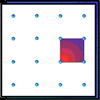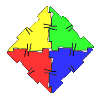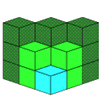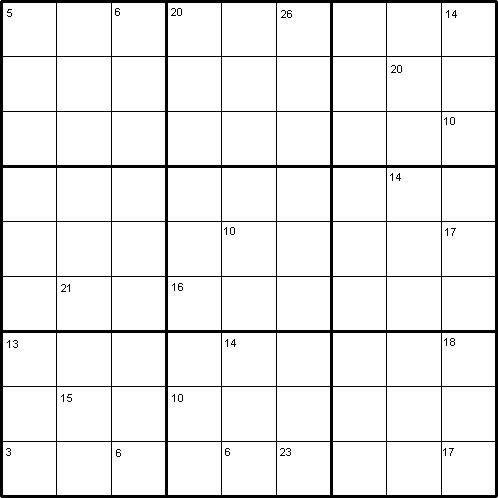#### You may also like### Geoboards

This practical challenge invites you to investigate the different squares you can make on a square geoboard or pegboard.### Polydron

This activity investigates how you might make squares and pentominoes from Polydron.If you had 36 cubes, what different cuboids could you make?

# Intersection Sums Sudoku

##### Age 7 to 16Challenge Level

by Henry Kwok### The Rules of "Intersection Sums Sudoku"

Like the standard Sudoku, this Sudoku variant consists of a grid of nine rows and nine columns subdivided into nine 3$\times$3 subgrids. Like the standard Sudoku, it has two basic rules:
1. Each column, each row, and each box (3$\times$3 subgrid) must have the numbers 1 to 9.
2. No column, row or box can have two squares with the same number.
Like other Sudokus published by NRICH, this puzzle can be solved with the help of the numbers in the top parts of certain squares. These numbers are the sums of the digits in all the squares horizontally and vertically adjacent to the square.

#### A Short Demonstration

The square in the bottom left corner of this Sudoku contains the number 3. 3 is the sum of the digits in the two adjacent squares, which therefore must contain the digits 1 and 2.
In the beginning, we do not know whether we should put 1 or 2 in the square (8,1) or in the square (9,2). If we put 1 in the square (9,2) and 2 in the square (8,1), we have to put 3 in the square (8,3) and 2 in the square (9,4) because of the small clue-number 6 in the square (9,3). If we put 2 in the square (9,2) and 1 in the square (8,1), we still have to put 3 in the square (8,3) and 1 in the square (9,4). We find that 3 will go to the square (8,3) regardless of where we put the rest of the numbers.

At least the answer for one square is confirmed. That's not too bad after all. Sooner or later, we shall be able to obtain the answers for the squares (8,1), (9,2) and (9,4) as we try to solve the rest of the puzzle.